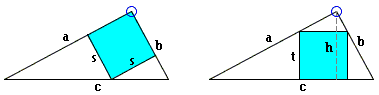## A Stronger Triangle Inequality

In 1997, a retired engineer (H. R. Bailley) and a retired chemist (R. Bannister) published a curious result - a strengthened triangle inequality - that serendipitously incorporated a rather ubiquitous consumer services symbol (24/7). A short while later, the now late math problem solver extraordinaire, Professor Murray Klamkin, gave an elegant 1 page proof of that result. I'll start with the motivation that drove Bailley and Bannister and proceed with Klamkin's proof.

Bailley and Bannister began there investigation with a right triangle and an inscribed square. They compared two ways of inscribing a square into a right triangle. Which of the squares is bigger?

### This applet requires Sun's Java VM 2 which your browser may perceive as a popup. Which it is not. If you want to see the applet work, visit Sun's website at https://www.java.com/en/download/index.jsp, download and install Java VM and enjoy the applet.

Denote the legs of the triangle as a and b, the hypotenuse c, the altitude to the hypotenuse h, and the sides of the two squares s and t.In both cases, the presence of similar triangles leads to the proportions:

 (b - s) / s = b / a and (c - t) / t = c / h,

from which

 b / s = (a + b) / a and c / t = (c + h) / h,

and ultimately

 s = ab / (a + b) and t = hc / (c + h).

Since, in a right triangle, both ab and hc equal double the area of the triangle, ab = hc. It is obvious then that the relative sizes of the squares inversely depend on the relative magnitudes of (a + b) and (c + h). To compare the two, consider

 (a + b)2 - (c + h)2 = (a2 + 2ab + b2) - (c2 + 2hc + h2) = (a2 + b2 - c2) + (2ab - 2hc) - h2 = - h2.

It follows that in a right triangle we always have

 (1) a + b < c + h.

Which is equivalent to s > t. Thus addition of the altitude h led to the inversion of the triangle inequality: a + b > c. Naturally, Bailley and Bannister decided to proceed and compare (a + b) and (c + h) for triangles other than right. They came up with a surprising result.

In a triangle with side length a, b, c, altitude h and the angle C opposite side c

 (2) a + b > c + h.

provided

or, as Bailley and Bannister put it, (2) holds for most triangles with C < π/2. Indeed arctan(24/7) is about 74°.

The proof below is due to M. Klamkin and is based on an identity

 (4) a + b - c = 2h·cos(C/2) / (cos (A-B)/2 + sin C/2),

where A and B are the angles in the triangle opposite a and b, respectively. (4) holds in any triangle.

For fixed C the ratio 2·cos(C/2) / (cos (A-B)/2 + sin C/2) clearly takes on its minimum when A = B (the triangle is isosceles), and this minimum is

 2·cos(C/2) / (1 + sin C/2) = 2(sec C/2 - tan C/2).

Thus the sharp triangle inequality

 a + b - c ≥ 2(sec C/2 - tan C/2)·h

is always valid, with equality if and only if a = b.

For (2) to hold we must have 2·cos(C/2) / (1 + sin C/2) > 1. The left side is a decreasing function of C and equals 1 when sin C/2 = 3/5, which corresponds to C = arctan(24/7). Thus (2) indeed holds for all C < arctan(24/7).

It remains only to prove (4). If R is the circumradius of the triangle,

 a = 2R·sin A, b = 2R·sin B, c = 2R·sin C, and h = a·sin B,

so that

 a + b - c = (sin A + sin B - sin C) / sin(A)sin(B).

Using elementary trigonometric identities and C = π - (A + B), the latter ratio becomes

 4·sin (A+B)/2 (cos (A-B)/2 - cos (A+B)/2) / (cos(A-B) - cos(A+B)) =       2·sin (A+B)/2 / (cos (A-B)/2 + cos (A+B)/2).

Using (A+B)/2 = π/2 - C/2, the ratio takes the form

 2·cos C/2 / (cos (A-B)/2 + sin C/2),

which is (4).

### References

1. H. R. Bailley, R. Bannister, A Stronger Triangle Inequality, The College Mathematics Journal, vol. 28, no. 3, May 1997, 182-186.
2. M. S. Klamkin, A Sharp Triangle Inequality, The College Mathematics Journal, vol. 29, no. 1, January 1998, 33.